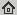# Numerical aspects of TV flow

Numerical Algorithms, 41(1): 79-101, Jan. 2006
Abstract: The singular diffusion equation called total variation (TV) flow plays an important role in image processing and appears to be suitable for reducing oscillations in other types of data. Due to its singularity for zero gradients, numerical discretizations have to be chosen with care. We discuss different ways to implement TV flow numerically, and we show that a number of discrete versions of this equation may introduce oscillations such that the scheme is in general not TV-decreasing. On the other hand, we show that TV flow may act self-stabilising: even if the total variation increases by the filtering process, the resulting oscillations remain bounded by a constant that is proportional to the ratio of mesh widths. For our analysis we restrict ourselves to the one-dimensional setting.

## BibTex reference

```@Article{Bro06d,
author       = "M. Breuß and T. Brox and A. B{\"u}rgel and T. Sonar and J. Weickert",
title        = "Numerical aspects of TV flow",
journal      = "Numerical Algorithms",
number       = "1",
volume       = "41",
pages        = "79-101",
month        = "Jan.",
year         = "2006",
url          = "http://lmb.informatik.uni-freiburg.de/Publications/2006/Bro06d"
}
```